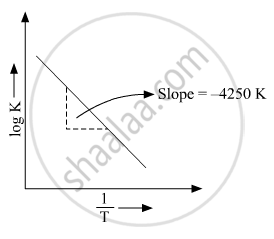# Rate Constant ‘K’ of a Reaction Varies with Temperature ‘T’ According to the Equation - Chemistry

(b) Rate constant ‘k’ of a reaction varies with temperature ‘T’ according to the equation:

logk=logA-E_a/2.303R(1/T)

Where Ea is the activation energy. When a graph is plotted for logk Vs. 1/T a straight line with a slope of −4250 K is obtained. Calculate ‘Ea’ for the reaction.(R = 8.314 JK−1 mol−1)

#### Solution

log k=logA-E_a/2.303R(1/T)

Ea → Activation energy

The above equation is like y = mx + c where if we plot y v/s x we get a straight line with slope ‘m’ and intercept ‘c’.So, slope is equal to=-E_a/2.303R

-E_a/2.303R=-4250k=>E_a=4250 xx 2.303 xx 8.314 =81,375.3535 j mol^(-1)

E_a=81.3753 KJ mol^(-1)

Concept: Temperature Dependence of the Rate of a Reaction
Is there an error in this question or solution?